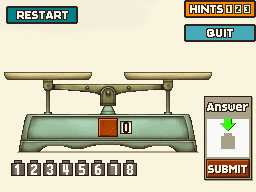### 006 Light WeightWalk rightExamine the window to the right of the brown door,
the sack hanging from the rightmost window
and the sidewalk sign on the left for hint coinsTalk to Marco

Puzzle 006Hint 1Hint 2Hint 3SolutionStart with Weighing 1

Weighing 1Weigh 1 2 3 on the left and 4 5 6 on the right

If the weights are even go to Weighing 2a
If the left side is lighter go to Weighing 2b
If the right side is lighter go to Weighing 2c

Weighing 2aWeigh 7 on the left and 8 on the right

If the left side is lighter then weight 7 is the answer
If the right side is lighter then weight 8 is the answer

Weighing 2bWeigh 1 on the left and 2 on the right

If the weights are even then weight 3 is the answer
If the left side is lighter then weight 1 is the answer
If the right side is lighter then weight 2 is the answer

Weighing 2cWeigh 4 on the left and 5 on the right

If the weights are even then weight 6 is the answer
If the left side is lighter then weight 4 is the answer
If the right side is lighter then weight 5 is the answer

Submit TipDrag the lightest weight to the answer box before tapping submit

Progress145 Picarats and 19 Hint Coins

005 Digital Digits (US)
005 Clock Hands (UK)